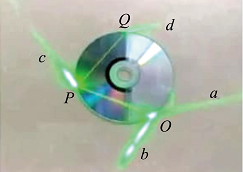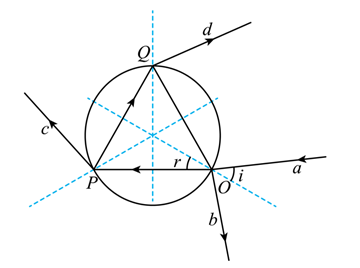$\text{A.}$ 光盘材料的折射率 2 $\text{B.}$ 光在光盘内的速度为真空中光速的三分之二 $\text{C.}$ 光束b、c和d的强度之和等于光束a的强度 $\text{D.}$ 光束c的强度小于O点处折射光束 的强度

D

#### 解析：A. 如图所示由几何关系可得入射角为
$$i=45^{\circ}$$

$$r=30^{\circ}$$

$$n=\frac{\sin 45^{\circ}}{\sin 30^{\circ}}=\frac{\frac{\sqrt{2}}{2}}{\frac{1}{2}}=\sqrt{2}$$

B. 根据
$$v=\frac{c}{n}=\frac{\sqrt{2}}{2} c$$

C. 光束在 $b 、 c$ 和 $d$ 的强度之和小于光束 $a$ 的强度, 因为在 $Q$ 处光还有反射光线, 所以 C 错误;
D. 光束 $c$ 的强度与反射光线 $P Q$ 强度之和等于折身光线 $O P$ 的强度，所以 $\mathrm{D}$ 正确;

①点击 收藏 此题， 扫码注册关注公众号接收信息推送（一月四份试卷,中1+高2+研1）
② 程序开发、服务器资源都需要大量的钱，如果你感觉本站好或者受到到帮助，欢迎赞助本站,赞助方式：微信/支付宝转账到 18155261033

 ①此题难易度如何 ②此题推荐度如何 确定